Sergey Horban is a QA Engineer with 3+ years of experience in automation and manual testing, bug-tracking and test management.

Learn JMeter in 5 Hours

Start Learning

Apr 01 2018

# How to Use Big Numbers in JMeter Scripts

As we already know from the previous article “Creating JMeter Variables in Java - The Ultimate Guide”, all primitive numeric data types have a limited character length, which is sometimes not long enough to perform, store and display mathematical calculations. If there are not enough primitive data types to represent numbers, then Big Numbers are used. Big numbers are numbers that consist of an arbitrary number of digits.

To work with large numbers, you can use the following classes in the java.math package:

• The BigDecimal class is used to perform calculations with real numbers of any length and precision.
• The BigInteger class is used to perform calculations with integers of any length.

In the practice of load testing, it is most common to encounter numbers that are represented by the BigDecimal class, so this is the class we will focus on in this blog post. Such numbers are used in any financial calculation, because you can clearly indicate the accuracy and type of rounding. In other words, if we are testing an API in which financial calculations are performed, then the numbers represented by the BigDecimal class are used.

In the previous article, “Mathematical Functions and Converting Data Types in Groovy”, we already said that in order to test any mathematical calculation that the API performs, we need to perform this calculation ourselves and compare it with the result that the API returns. In this article, we will focus on how to create variables with the BigDecimal data type in Apache JMeter™ and how to perform mathematical operations with such variables.

To create a variable with the BigDecimal data type, you need to do the following: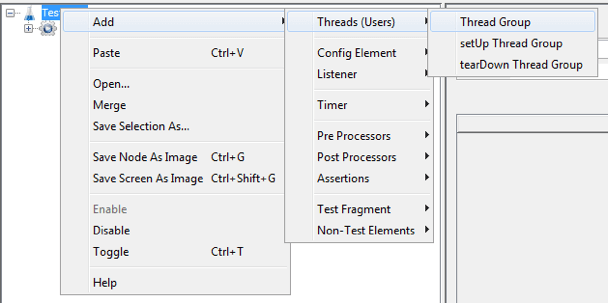Thread Group -> Right Click -> Add -> Sampler -> JSR223 Sampler

JSR223 Sampler -> Language Groovy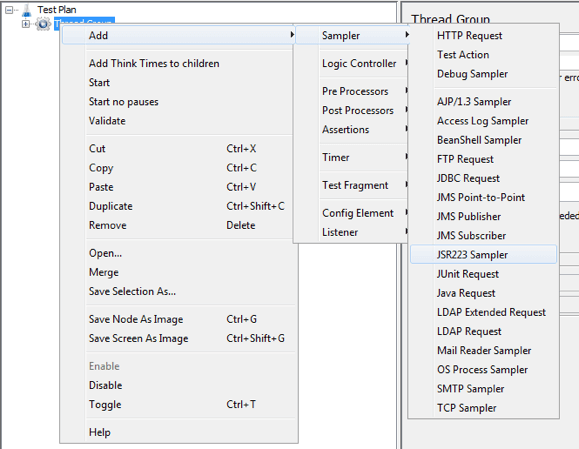At this step, we will create numbers represented by the BigDecimal class.

In the JSR223 Sampler, add the following code example.

```int a = 23;
long b = 12345678;
double c = 23.123;
String d = "23.333333344445555566666600034758866";

BigDecimal bigDecimalFromInt = new BigDecimal(a);
BigDecimal bigDecimalFromLong = new BigDecimal(b);
BigDecimal bigDecimalFromDouble = new BigDecimal(c);
BigDecimal bigDecimalFromString = new BigDecimal(d);

log.info(bigDecimalFromInt + "");
log.info(bigDecimalFromLong + "");
log.info(bigDecimalFromDouble + "");
log.info(bigDecimalFromString + "");
```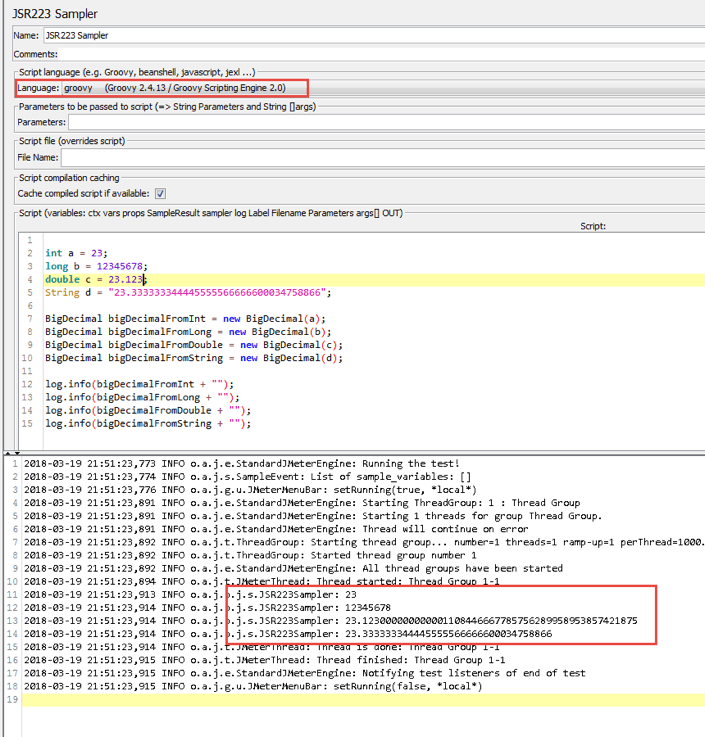int a = 23;
long b = 12345678;
double c = 23.123;
String d = "23.333333344445555566666600034758866";
- Creating variables with a primitive data type.

BigDecimal bigDecimalFromInt = new BigDecimal(a); - Creating a variable with the BigDecimal data type using a variable with data type int

BigDecimal bigDecimalFromLong = new BigDecimal(b); - Creating a variable with the BigDecimal data type using a variable with data type long

BigDecimal bigDecimalFromDouble = new BigDecimal(c); - Creating a variable with the BigDecimal data type using a variable with data type double

BigDecimal bigDecimalFromString = new BigDecimal(d); - Creating a variable with the BigDecimal data type using a variable with data type string

log.info(bigDecimalFromInt + ""); - A method that outputs data to the console. In our case, this method outputs the value of “bigDecimalFromInt, bigDecimalFromLong, bigDecimalFromDouble, bigDecimalFromString” to the console.

As you can see in the image above, the console shows the result of creating Big Numbers.

To create a variable with the BigDecimal data type, you can use not only variables, but also corresponding literals (in our case, the literals will be the following values: 23, 234567811111111111233322233333331111111111111111111, 23.123, 23.333333344445555566666600034758866), as shown below.

In the JSR223 Sampler, add the following code example:

```BigDecimal bigDecimalFromInt = new BigDecimal(23);

BigDecimal bigDecimalFromLong = new BigDecimal(12345678111111111112333222333333311111111111111111);

BigDecimal bigDecimalFromDouble = new BigDecimal(23.123);

BigDecimal bigDecimalFromString = new BigDecimal("23.333333344445555566666600034758866");

log.info(bigDecimalFromInt + "");
log.info(bigDecimalFromLong + "");
log.info(bigDecimalFromDouble + "");
log.info(bigDecimalFromString + "");
```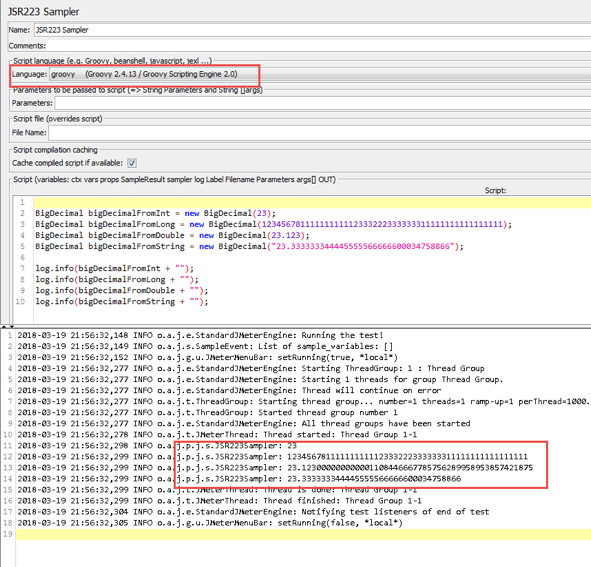In the image above, the console shows the result of creating Big Numbers.

When creating variables with the BigDecimal data type, you can set the following parameters:

• The number of characters that must contain a value (for example, we can specify that our value consists of 4 characters (23.33) or 5 characters (12.234) )
• The number of characters after the floating point
• The rounding method

For variables of BigDecimal type, the following rounding rules exist:

• BigDecimal.ROUND_CEILING or RoundingMode.CEILING - rounding up
• BigDecimal.ROUND_FLOOR or RoundingMode.FLOOR - rounding down
• BigDecimal.ROUND_UP or RoundingMode.UP  - rounding up by the number modulus
• BigDecimal.ROUND_DOWN  or RoundingMode.DOWN  - rounding down by the number modulus
• BigDecimal.ROUND_HALF_DOWN or RoundingMode.HALF_DOWN  -  If the number after the floating point is greater than 5, then rounding up is performed
• BigDecimal.ROUND_HALF_EVEN or RoundingMode.HALF_EVEN - If rounding is performed for the number 5 and an even number is on the left, then rounding down is performed. If rounding is performed for the number 5 and there is not an even number on the left, then rounding up is performed
• BigDecimal.ROUND_HALF_UP or RoundingMode.HALF_UP -  rounding up is performed if the number after floating point > = 5
• BigDecimal.ROUND_UNNECESSARY  or RoundingMode.UNNECESSARY -  This rule is used only for integer values. Indicates that rounding does not need to be performed

Given this, let’s look at how the variables of the BigDecimal type can be created.

In the JSR223 Sampler, add the following code example.

```import java.math.MathContext;
import java.math.RoundingMode;

double a = 23.22;
double a1 = 23;

BigDecimal bigDecimal = new BigDecimal(a,new MathContext(10));
BigDecimal bigDecimal1 = new BigDecimal(a1,new MathContext(10));
BigDecimal bigDecimal2 = new BigDecimal(a,new MathContext(10,RoundingMode.DOWN));

log.info(bigDecimal + "");
log.info(bigDecimal1 + "");
log.info(bigDecimal2 + "");
log.info("");

BigDecimal bigDecimal3 = new BigDecimal(a).setScale(6,BigDecimal.ROUND_DOWN);
BigDecimal bigDecimal4 = new BigDecimal(a).setScale(6,1);

log.info(bigDecimal3 + "");
log.info(bigDecimal4 + "");
log.info("");

BigDecimal bigDecimal5 = new BigDecimal(a,new MathContext(10,RoundingMode.DOWN)).setScale(3,BigDecimal.ROUND_UP);
log.info(bigDecimal5 + "");
```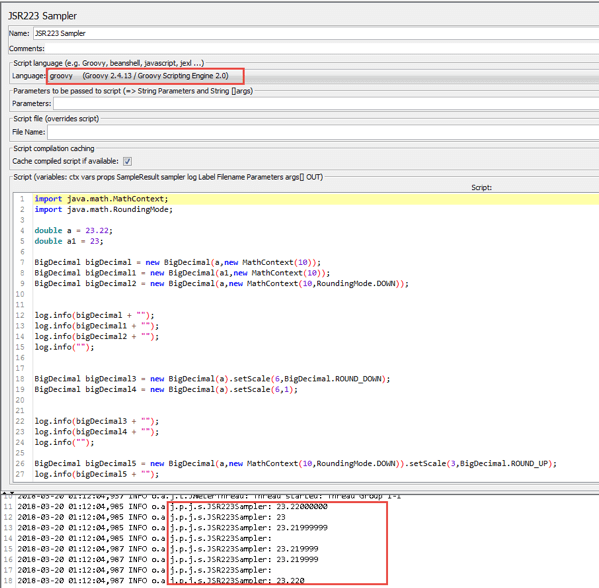double a = 23.22;
double a1 = 23;
- Creating variables with data type double

BigDecimal bigDecimal = new BigDecimal(a,new MathContext(10)); - Create a variable with a BigDecimal data type using a variable with a double data type.

new MathContext (10) - Sets the total number of signs/characters after the floating point, taking into account the signs of the integer part.

10 - the number of signs after the floating point, taking into account the signs of the integer part. In our case, for 23.22, 8 signs are set after the floating point, since the integer part already has 2 signs. If, for example, the integer part has 3 signs, then after the floating point, 7 signs will be set.

If the value contains signs after the floating point, but does not contain the number of signs after the floating point that new MathContext (10) sets, then these values ​​are set to "0". In our case, the variable "a" after the floating point has only 2 signs, respectively, the remaining 6 signs are set to "0"

BigDecimal bigDecimal1 = new BigDecimal(a1,new MathContext(10)); - Create a variable with a BigDecimal data type using a variable with a double data type. In this variant, it is shown that if the value has only the integer part, but new MathContext (10) is used, then "0" is not added. It remains only the integer part

BigDecimal bigDecimal2 = new BigDecimal(a,new MathContext(10,RoundingMode.DOWN)); - This variant is similar to the previous one, but adds RoundingMode.DOWN. RoundingMode.DOWN is a rounding rule. If we do not pass a rounding rule, as it was in the example above, then the default value is set to RoundingMode.HALF_UP (Rounding up if the number after the decimal point is >= 5)

In the examples above, when we created a variable with the type BigDecimal in the following form: BigDecimal bigDecimalFromInt = new BigDecimal (a); or BigDecimal bigDecimalFromInt = new BigDecimal (23);, we did not use new MathContext (10, RoundingMode.DOWN). In this case, the default values that are set are: BigDecimal bigDecimalFromInt = new BigDecimal (a, new MathContext (0, RoundingMode.HALF_UP)), where "0" is an unlimited number of signs.

BigDecimal bigDecimal3 = new BigDecimal(a).setScale(6,BigDecimal.ROUND_DOWN); - setScale(6,BigDecimal.ROUND_DOWN) - A method that sets the number of signs after a floating point. In our case, 6 signs are set (23.219999). BigDecimal.ROUND_DOWN - rounding rule.

BigDecimal bigDecimal4 = new BigDecimal(a).setScale(6,1);  - setScale (6,1) - A method that sets the number of signs after a floating point. In our case, 6 signs are set (23.219999).
1 is the equivalent of the next BigDecimal.ROUND_DOWN entry. In other words, we can pass a rounding rule to the setScale () method, for example BigDecimal.ROUND_DOWN or the corresponding numeric value.

The corresponding numbers that are shown below are equivalent to rounding rules and we can use them instead of writing the rounding rule.

 Rounding Rule Equivalent Numerical Value BigDecimal.ROUND_UP 0 igDecimal.ROUND_DOWN 1 BigDecimal.ROUND_CEILING 2 BigDecimal.ROUND_FLOOR 3 BigDecimal.ROUND_HALF_UP 4 BigDecimal.ROUND_HALF_DOWN 5 BigDecimal.ROUND_HALF_EVEN 6 BigDecimal.ROUND_UNNECESSARY 7

BigDecimal bigDecimal5 = new BigDecimal(a,new MathContext(10,RoundingMode.DOWN)).setScale(3,BigDecimal.ROUND_DOWN); - With this variant of creating a variable, only the values that are passed to the setScale () method will be set. In our case, this is 3 signs after the floating point and the rounding rule BigDecimal.ROUND_UP (rounding up in modulus).

If we use the setScale () method, then we must specify the rounding rule, otherwise we will get an error, as shown in the image below.

In the JSR223 Sampler, add the following code example:

```import java.math.MathContext;
import java.math.RoundingMode;

double a = 23.22;
double a1 = 23;

BigDecimal bigDecimal3 = new BigDecimal(a).setScale(6);
```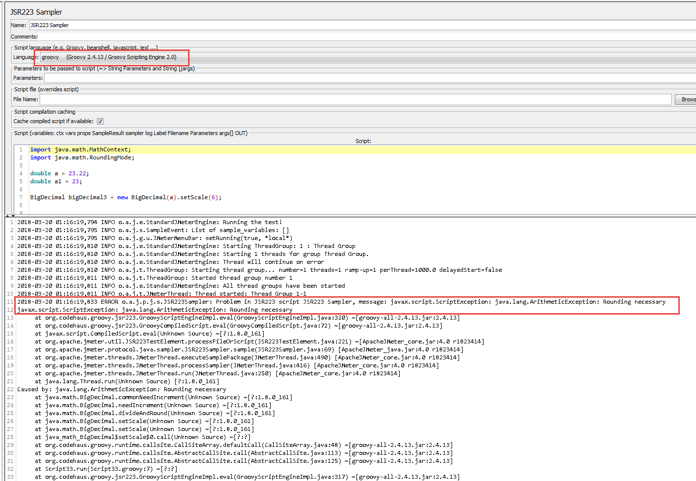The image above shows an error that occurs if you do not specify a rounding rule in the setScale () method.

Below you will see how the rounding rules work for variables with the BigDecimal data type.

In the JSR223 Sampler, add the following code example:

```import java.math.MathContext;
import java.math.RoundingMode;

double c1 = -23.2332;
double c2 = 23.2332;

//Rounding up is performed always

BigDecimal bigDecimal1 = new BigDecimal(c1).setScale(3,BigDecimal.ROUND_CEILING);
BigDecimal bigDecimal2 = new BigDecimal(c2).setScale(3,BigDecimal.ROUND_CEILING);

log.info("ROUND_CEILING: " + bigDecimal1);
log.info("ROUND_CEILING: " + bigDecimal2);

//Rounding down is performed always

BigDecimal bigDecimal3 = new BigDecimal(c1).setScale(3,BigDecimal.ROUND_FLOOR);
BigDecimal bigDecimal4 = new BigDecimal(c2).setScale(3,BigDecimal.ROUND_FLOOR);

log.info("ROUND_FLOOR: " + bigDecimal3);
log.info("ROUND_FLOOR: " + bigDecimal4);

//The modulus of the number is determined, and then the rounding up is always performed for the resulting module.

BigDecimal bigDecimal5 = new BigDecimal(c1).setScale(3,BigDecimal.ROUND_UP);
BigDecimal bigDecimal6 = new BigDecimal(c2).setScale(3,BigDecimal.ROUND_UP);

log.info("ROUND_UP: " + bigDecimal5);
log.info("ROUND_UP: " + bigDecimal6);

//The modulus of the number is determined, and then the rounding down is always performed for the resulting module.

BigDecimal bigDecimal7 = new BigDecimal(c1).setScale(3,BigDecimal.ROUND_DOWN);
BigDecimal bigDecimal8 = new BigDecimal(c2).setScale(3,BigDecimal.ROUND_DOWN);

log.info("ROUND_DOWN: " + bigDecimal7);
log.info("ROUND_DOWN: " + bigDecimal8);

// If the number after the floating point is greater than 5, then rounding up is performed in the large side

double c3 = 23.6;
double c4 = 23.5;
double c5 = 23.4;

BigDecimal bigDecimal9 = new BigDecimal(c3).setScale(0,BigDecimal.ROUND_HALF_DOWN);
BigDecimal bigDecimal10 = new BigDecimal(c4).setScale(0,BigDecimal.ROUND_HALF_DOWN);

log.info("ROUND_HALF_DOWN: " + bigDecimal9);
log.info("ROUND_HALF_DOWN: " + bigDecimal10);

// If the number after the floating point is greater than 5 or equals 5, then rounding up is performed in the large side

BigDecimal bigDecimal11 = new BigDecimal(c3).setScale(0,BigDecimal.ROUND_HALF_UP);
BigDecimal bigDecimal12 = new BigDecimal(c4).setScale(0,BigDecimal.ROUND_HALF_UP);
BigDecimal bigDecimal13 = new BigDecimal(c5).setScale(0,BigDecimal.ROUND_HALF_UP);

log.info("ROUND_HALF_UP: " + bigDecimal11);
log.info("ROUND_HALF_UP: " + bigDecimal12);
log.info("ROUND_HALF_UP: " + bigDecimal13);

// If rounding is performed for the number 5 and an even number is on the left, then rounding down is performed
// If rounding is performed for the number 5 and there is not an even number on the left, then rounding up is performed

double c6 = 23.25;
double c7 = 23.35;

BigDecimal bigDecimal14 = new BigDecimal(c6).setScale(1,BigDecimal.ROUND_HALF_EVEN);
BigDecimal bigDecimal15 = new BigDecimal(c7).setScale(1,BigDecimal.ROUND_HALF_EVEN);

log.info("ROUND_HALF_EVEN: " + bigDecimal14);
log.info("ROUND_HALF_EVEN: " + bigDecimal15);

//This rule is used only for integer values. Indicates that rounding does not need to be performed.

double c8 = 23;
BigDecimal bigDecimal16 = new BigDecimal(c8).setScale(4,BigDecimal.ROUND_UNNECESSARY);

log.info("ROUND_UNNECESSARY: " + bigDecimal16);
```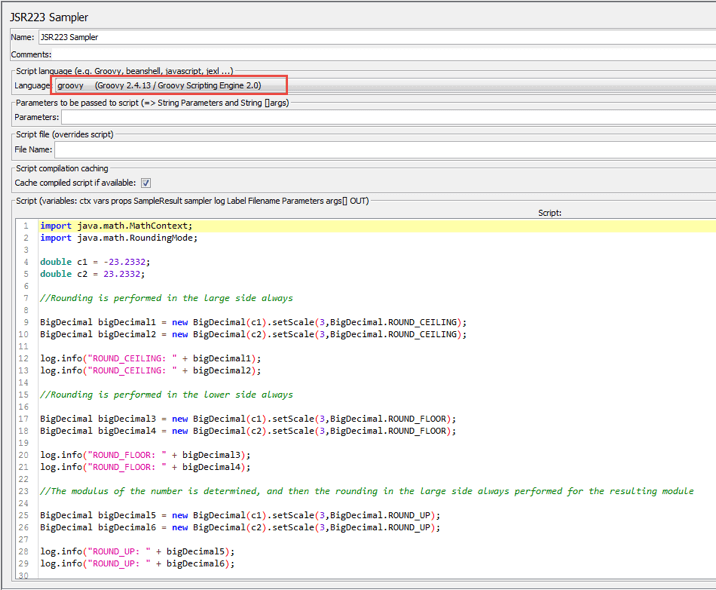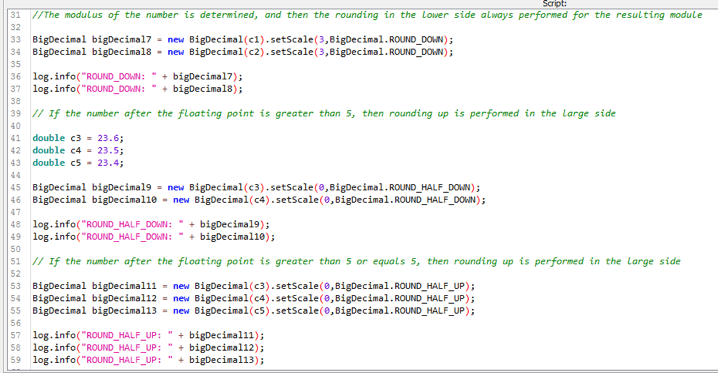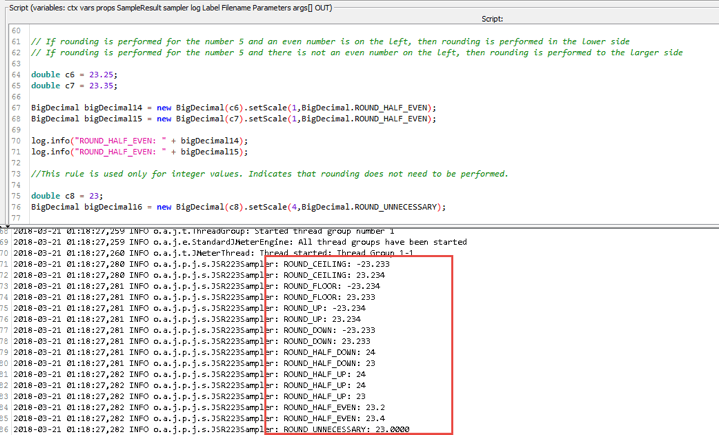To perform the mathematical operations of multiplying and dividing variables with the BigDecimal data type, there are appropriate methods. The arithmetic operations “*” and “/” with BigDecimal are not used and lead to an error.

In order to perform arithmetic operations with BigDecimal, you need to do the following:

In the JSR223 Sampler, add the following code example:

```import java.math.MathContext;
import java.math.RoundingMode;

double c1 = -23;
double c2 = 12;
double c3 = 3;
double c4 = 3;

BigDecimal bigDecimal1 = new BigDecimal(c1).abs();
log.info("abs: " + bigDecimal1);

BigDecimal bigDecimal2 = new BigDecimal(c2).divide(new BigDecimal(c3));
log.info("divide: " + bigDecimal2);

BigDecimal bigDecimal3 = new BigDecimal(c2).multiply(new BigDecimal(c3));
log.info("multiply: " + bigDecimal3);

BigDecimal bigDecimal4 = new BigDecimal(c2) + new BigDecimal(c3);
log.info("+ : " + bigDecimal4);

BigDecimal bigDecimal5 = new BigDecimal(c2) - new BigDecimal(c3);
log.info("- : " + bigDecimal5);

int a = new BigDecimal(c2).compareTo(new BigDecimal(c3));
int a1 = new BigDecimal(c3).compareTo(new BigDecimal(c2));
int a2 = new BigDecimal(c3).compareTo(new BigDecimal(c4));

log.info("compareTo: " + a);
log.info("compareTo: " + a1);
log.info("compareTo: " + a2);
```• abs() - A method that returns the absolute value of a variable with a BigDecimal data type
• divide() - A method that divides two variables with a BigDecimal data type
• multiply() - A method that performs multiplication of two variables with the BigDecimal data type
• “+” - Addition of two variables with data type BigDecimal
• “-” - Subtraction of two variables with the BigDecimal data type
• int a = new BigDecimal(c2).compareTo(new BigDecimal(c3)); - A method that compares two variables with the BigDecimal data type. If the value to the left of compareTo is greater than the value on the right, then the method returns "1"
• int a1 = new BigDecimal(c3).compareTo(new BigDecimal(c2)); - If the value to the left of compareTo is less than the value on the right, then the method returns "-1"
• int a2 = new BigDecimal(c3).compareTo(new BigDecimal(c4)); - If the value to the left of compareTo is equal to the value on the right, then the method returns "0"

As you can see, the BigDecimal class contains a fairly large number of methods for performing various mathematical operations, which will be very useful in developing tests. To learn more advanced JMeter, go to our free JMeter academy.

Run your JMX scripts in BlazeMeter and get collaboration, scalability, advanced reporting and tests from multiple geo-locations. To try us out, request a demo, or put your URL in the box below and your test will start in minutes.

### You might also find these useful:

Interested in writing for our Blog?Send us a pitch!

Your email is required to complete the test. If you proceed, your test will be aborted.